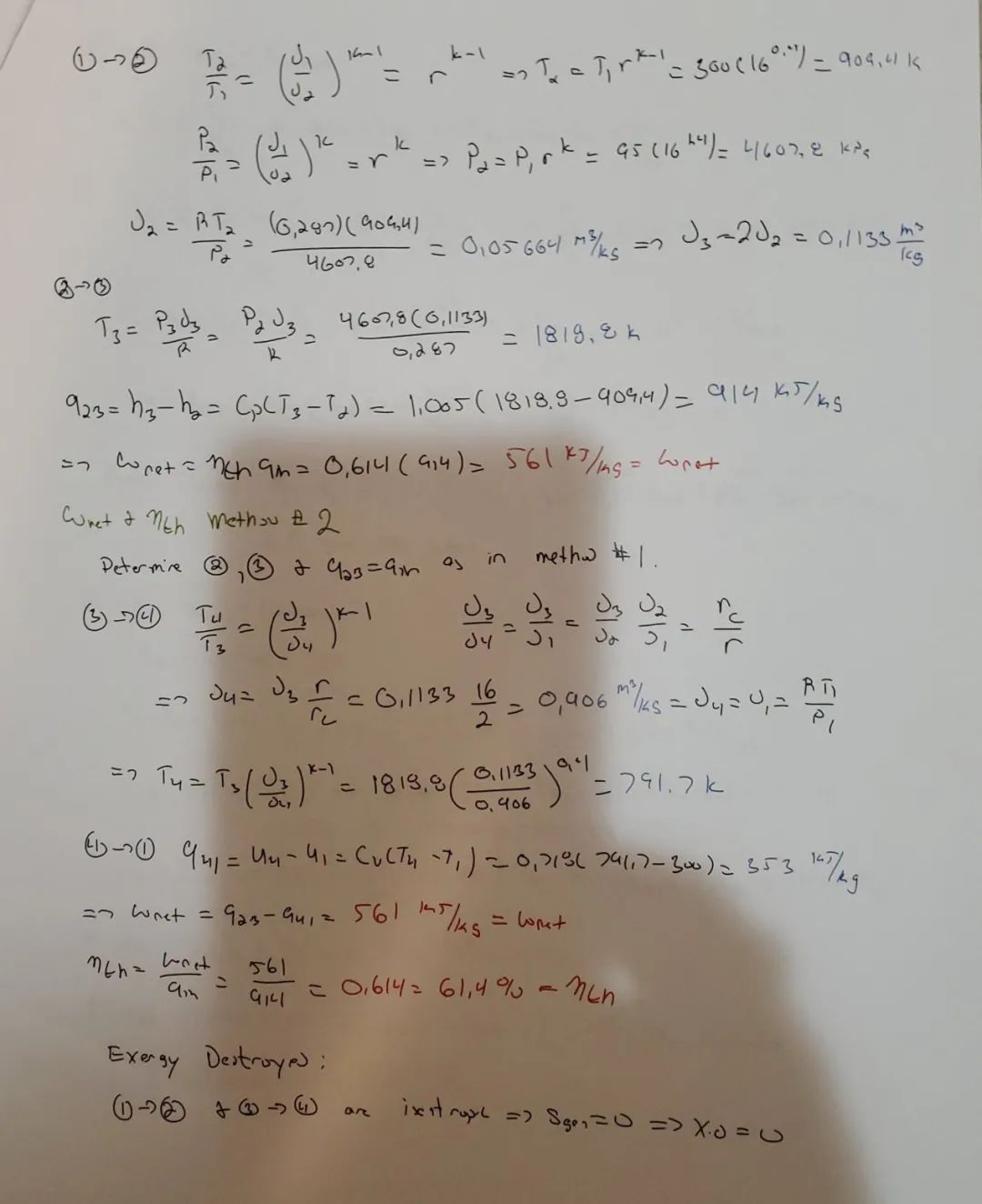Test_image``````import cv2
import numpy as np
import matplotlib.pyplot as plt
``````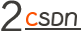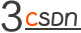• max_filtering（）函数接受输入图像和窗口大小 N；

• 它最初在输入数组周围创建一个“墙”（带有-1的填充），当我们遍历边缘像素时会有所帮助；

• 然后，我们创建一个“ temp”变量，将计算出的最大值复制到其中；

• 然后，我们遍历该数组并围绕大小为 N x N 的当前像素创建一个窗口；

• 然后，我们使用“ amax（）”函数在该窗口中计算最大值，并将该值写入 temp 数组；

• 我们将该临时数组复制到主数组 A 中，并将其作为输出返回。

• A 是输入 I 的最大滤波图像。

``````def max_filtering(N, I_temp):
wall = np.full((I_temp.shape+(N//2)*2, I_temp.shape+(N//2)*2), -1)
wall[(N//2):wall.shape-(N//2), (N//2):wall.shape-(N//2)] = I_temp.copy()
temp = np.full((I_temp.shape+(N//2)*2, I_temp.shape+(N//2)*2), -1)
for y in range(0,wall.shape):
for x in range(0,wall.shape):
if wall[y,x]!=-1:
window = wall[y-(N//2):y+(N//2)+1,x-(N//2):x+(N//2)+1]
num = np.amax(window)
temp[y,x] = num
A = temp[(N//2):wall.shape-(N//2), (N//2):wall.shape-(N//2)].copy()
return A
``````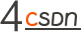``````def min_filtering(N, A):
wall_min = np.full((A.shape+(N//2)*2, A.shape+(N//2)*2), 300)
wall_min[(N//2):wall_min.shape-(N//2), (N//2):wall_min.shape-(N//2)] = A.copy()
temp_min = np.full((A.shape+(N//2)*2, A.shape+(N//2)*2), 300)
for y in range(0,wall_min.shape):
for x in range(0,wall_min.shape):
if wall_min[y,x]!=300:
window_min = wall_min[y-(N//2):y+(N//2)+1,x-(N//2):x+(N//2)+1]
num_min = np.amin(window_min)
temp_min[y,x] = num_min
B = temp_min[(N//2):wall_min.shape-(N//2), (N//2):wall_min.shape-(N//2)].copy()
return B
``````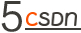``````#B is the filtered image and I is the original image
def background_subtraction(I, B):
O = I - B
norm_img = cv2.normalize(O, None, 0,255, norm_type=cv2.NORM_MINMAX)
return norm_img
``````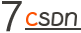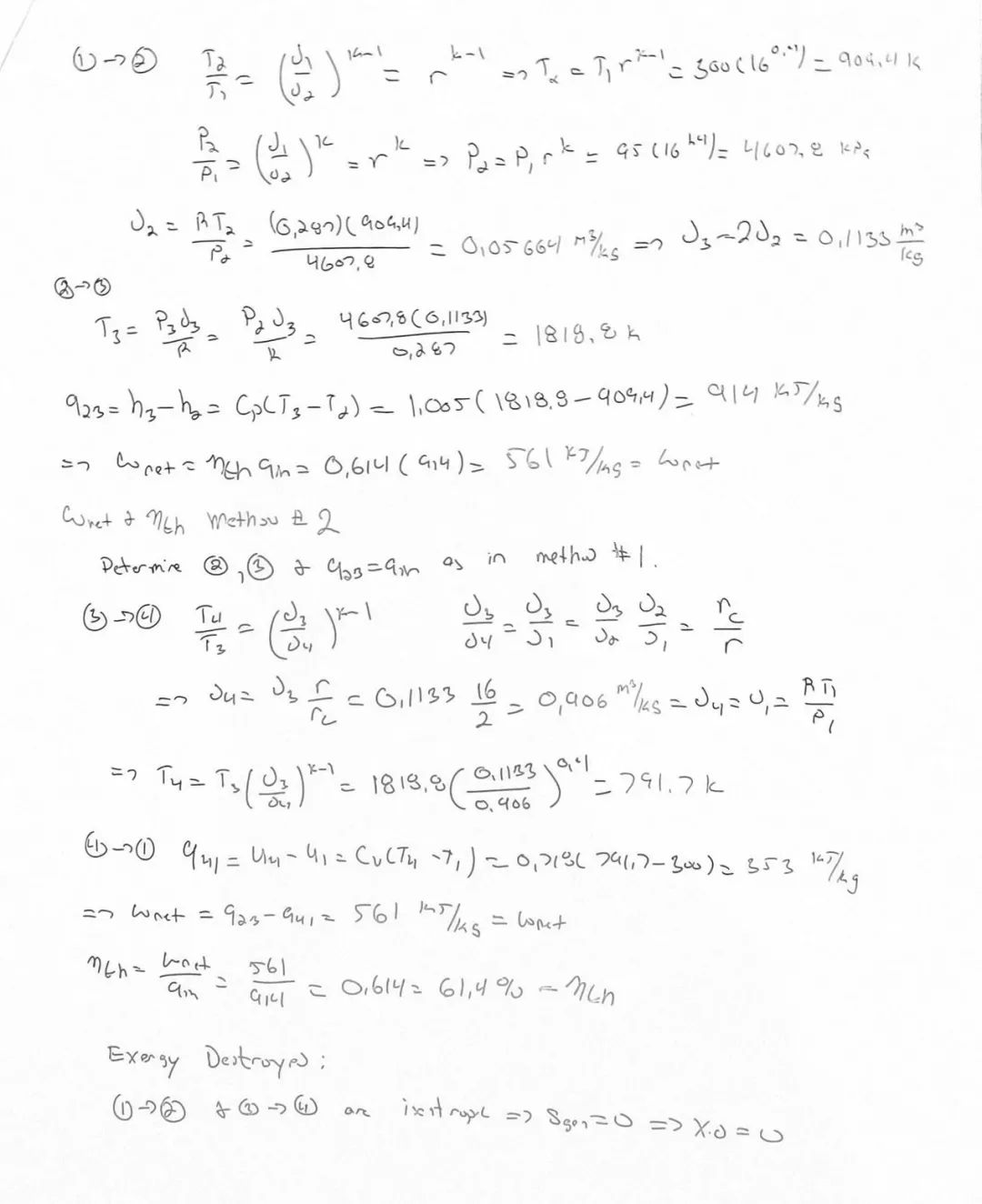Test_image_output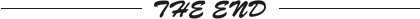``````更多精彩推荐
``````
``````☞红帽将停止支持 CentOS 8；2020 百度沸点揭晓年度知识热词；Qt 6.0 发布|极客头条
☞踢掉 Docker 后，Kubernetes 还能欢快地跑 GPU？
☞科技垄断正在朝着纵向发展

☞挑战TensorFlow、PyTorch，谁才是中国AI开源框架之星
☞被弃用的 Docker 会被 Podman 取代吗？
☞索要 2.3 亿元赎金！富士康遭遇黑客攻击

``````
``````点分享点点赞点在看
``````20年前，《新程序员》创刊时，我们的心愿是全面关注程序员成长，中国将拥有新一代世界级的程序员。20年后的今天，我们有了新的使命：助力中国IT技术人成长，成就一亿技术人！